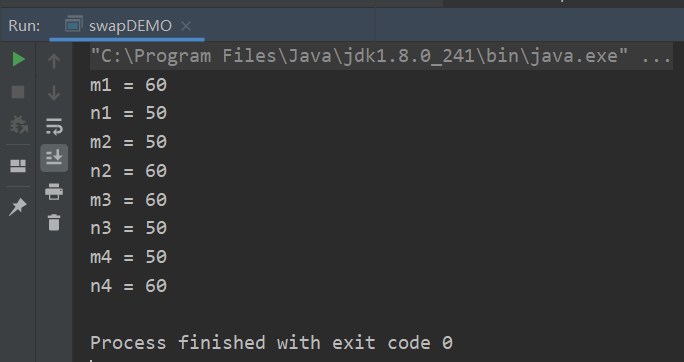• 1 加减法： // 方法一：该方法可以交换整型和浮点型数值的变量，但在处理浮点型的时候有可能出现精度的损失 int a=1; int b=2;  a = a + b; b = a - b; a = a - b; System.out.printf("a = %d\t,b =%d\n...
1 加减法： // 方法一：该方法可以交换整型和浮点型数值的变量，但在处理浮点型的时候有可能出现精度的损失
int a=1;     int b=2;             a = a + b;     b = a - b;     a = a - b;
System.out.printf("a = %d\t,b =%d\n",a,b);
2乘除法： //方法二：可以完成对整型变量的交换，对于浮点型变量它无法完成交换。
int a=1;     int b=2;     a = a * b;     b = a / b;     a = a / b;
System.out.printf("a = %d\t,b =%d\n",a,b);
3异或;//可以处理整型和浮点型变量，但在处理浮点型变量时也存在精度损失问题。而且乘除法比加减法要多一条约束：b必不为0。     int a = 1;     int b = 2;     a = a^b;     b = a^b;     a = a^b;    System.out.printf("a = %d\t,b =%d\n",a,b);     
展开全文• 使用临时变量交换2个变量的需求原理代码方法1（先+后-）方法2（先*后/）方法3（先-后+）方法4（位异或^）执行验证要点 需求 平时交换2个变量的都要用到临时变量。笔者突发奇想，使用临时变量是否依然可以...


不使用临时变量交换2个变量的值
需求原理代码方法1（先+后-）方法2（先*后/）方法3（先-后+）方法4（位异或^）执行验证要点

需求
平时交换2个变量的值都要用到临时变量。笔者突发奇想，不使用临时变量是否依然可以减缓2个变量的值？
原理
代码
使用c#和java实现的代码是相同的。笔者习惯使用C#做桌面上位机开发，使用java做算法验证。
public class swapDEMO {
public static void main(String[] args) {
int m = 50;
int n = 60;

//方法1
m += n;
n = m - n;
m -= n ;
System.out.println("m1 = " + m);
System.out.println("n1 = " + n);

//方法2
m *= n;
n = m / n;
m /= n;

System.out.println("m2 = " + m);
System.out.println("n2 = " + n);

//方法3
m -= n;
n += m;
m = n - m;

System.out.println("m3 = " + m);
System.out.println("n3 = " + n);

//方法4
m = m ^ n;
n = m ^ n;
m = m ^ n;

System.out.println("m4 = " + m);
System.out.println("n4 = " + n);

}
}


方法1（先+后-）
方法2（先*后/）
方法3（先-后+）
方法4（位异或^）
执行验证要点
笔者以交换整数为例。由于【+ - *】运算都是可逆的，【 / 】运算是不可逆的，故只能先 【 * 】后【 / 】不能先【 / 】后【 * 】。 使用位异或【 ^ 】的原理是任意数字连续2次^异或，得到的还是本身。 同理，正运算可以使用累乘运算，逆运算可以使用开方运算。但是计算机在进行开方等运算时会产生浮点数，造成精度丢失。故这种方式不可取。同理，指数对数做正逆运算的方式也不可取。
使用【±*】运算时要先确认所用数据类型是否能存储下计算的结果，要小心数据溢出。而异或^运算不存在数据溢出的问题，显然是最优解。
展开全文c# java 算法
• * 定义两个变量不定义第三个变量的情况下交换两个变量 */ public class jiaoHuan { public static void main(String[] args) { String s1 = "00"; String s2 = "abcd"; System....
/**
* 定义两个变量 在不定义第三个变量的情况下交换两个变量的值
*/
public class jiaoHuan {

public static void main(String[] args) {
String s1 = "00";
String s2 = "abcd";
System.out.println("交换之前 s1 = " + s1 + " s2 = " + s2);
s2 = s1 + s2; //合并到s2
System.out.println("s2 = " + s2); //00abcd
s1 = s2.substring(s1.length());
//截取前面长度为4的字符串 s1 = "abcd"
s2 = s2.substring(0, s2.length() - s1.length());
//截取最后长度为2的字符串 s2 = "00"
System.out.println("交换后 s1 = " + s1 + " s2 = " + s2);
}

}

/**
* int类型
*/
class testInt {

public static void main(String[] args) {
int a = 1;
int b = 9;
System.out.println("交换之前 a = " + a + " ，b = " + b);
a = a + b;
b = a - b;
a = a - b;
System.out.println("交换之后 a = " + a + " ,b = " + b);
}
}

展开全文Java面试题
• 面试中经常考到的一个题。原理跟 a=a+b;...//宏定义 交换两个变量。使用异或运算。 #define SWAP(a,b) {a=a^b; b=a^b; a=a^b;} int main() {  int i, j;  i = 1213;  j = 1314;  SWAP(i, j);  ...
面试中经常考到的一个题。原理跟 a=a+b;   b=a-b;  a=a-b;  一样的，很简单，不解释。

//宏定义 交换两个变量的值。使用异或运算。 #define SWAP(a,b) {a=a^b; b=a^b; a=a^b;}
int main() {     int i, j;     i = 1213;     j = 1314;
SWAP(i, j);     printf("i=%d,j=%d \n", i, j);
return 0;
}

展开全文C语言
• // 进行交换值操作 c = a; a = b; b = c; 如果采用上面的方法，我们必须要浪费掉一个临时变量，但是如果我们采用异或操作的话，就能直接利用这两个变量来实现，变量互换的操作。public Change {java
• 本文给出使用中间变量交换两个变量值得源码方法。swap
• 通常我们的做法是（尤其是在学习阶段）：定义一个变量，借助它完成交换。代码如下： int a,b; a=10; b=15; int t; t=a; a=b; b=t; 这种算法易于理解，特别适合帮助初学者了解计算机程序的特点，是赋值语句的经典...
• 引入第三变量交换变量值，其中最常用的就应该是位运算了： int a=10=1010,int b=12=1100, a=a^b;//1010^1100=0110 b=a^b;//0110^1100=1010 a=a^b;//0110^1010=1100; 此算法能够实现是由异或运算的特点决定的，...
• 通常我们的做法是（尤其是在学习阶段）：定义一个变量，借助它完成交换。代码如下： int a,b; a=10; b=15; int t; t=a; a=b; b=t; 这种算法易于理解，特别适合帮助初学者了解计算机程序的特点，是赋值语句的经典...算法 编译器 语言
•  学过编程的人都知道，在交换两个变量时，大多数人习惯定义一个临时变量来存放其中的一个，防止交换数值时其中一个变量被覆盖掉，比如下面的一段用C++写的很简单的程序： /**交换两个变量*/ #include...位运算 c++
• 我在写我的第一篇博客，想想还有点小激动呢，第一次写，可能写得尽如人意，还望大家多多谅解鸭，有什么正确的地方，还望大家指正。 今天看了一个大佬的代码，发现了一个这样的几行代码： arr[i]=arr[i]^arr...
• [转载地址]http://blog.csdn.net/q1056843325/article/details/53223914交换变量值方案一最最最简单的办法就是使用一个临时变量了 不过使用临时变量的方法实在是太low了var t; t = a; a = b; b = t;首先把a的存储...javascript 面试题
• 直接将a， b两个变量放到元组中，再通过元组按照index进行赋值的方式进行重新赋值给两个变量。 def demo1(a,b): a,b = b,a print(a,b) 方法二： 通过简单的逻辑运算进行将两个进行互换 def demo2(a, b): a = a...
• 方法1：实现两个变量值交换使用第三个变量 我们可以通过数学运算中的差值来实现交换   int a=10,b=20;     a=a+b;     b=a-b;     a=a-b;     交换成功，运行结果如下图 ...
• #include #include struct stuff{　char full_name;...void swap_struct_data(char *ptr_a, char *ptr_b, int size){/*函数：swap_struct_data作用：交换两个结构型变量参数：ptr_a 变量A的地址，ptr_struct function include
• 在解题过程中，你可能会经常遇到需要交换两个变量的时候，大部分的情况下，我们引入了一个变量，来帮助我们完成交换。...如何申请变量，而达到交换两个变量的目的。 提示一下
• 通常我们在进行两个变量交换这样的操作时，往往要引入第三个变量，借助第三个变量，交换两个变量的。这种方法是最为简单的，也是十分容易理解的，代码如下：（这里我们采用c++的形式表现，道理相同） #include ...c语言基础
• 给两个整型变量赋值，然后交换两个变量，最后输出交换后的变量值
• publicstaticvoidtest(inta,intb){...System.out.println("交换前 a="+a); System.out.println("交换前 b="+b); a=a+b; //a=3+5=8 b=a-b; //b=8-5=3 a=a-b; //a=8-3=5 System.out.println("交换后 a="+a); Sys...Java
• 通常我们的做法是（尤其是在学习阶段）：定义一个变量，借助它完成交换。代码如下： int a,b; a=10; b=15; int t; t=a; a=b; b=t; 这种算法易于理解，特别适合帮助初学者了解计算机程序的特点，是赋值语句...算法
• 第二种方法就是使用第三个变量的情况下交换两个变量值。 下面我们就通过具体的代码示例，给大家介绍PHP交换两个变量值的方法。 首先大家要知道交换两个变量就是指相互交换变量。通常，这是通过内存中的数据...
• 通常我们的做法是（尤其是在学习阶段）：定义一个变量，借助它完成交换。代码如下：  int a,b;  a=10; b=15;  int t;  t=a; a=b; b=t;  这种算法易于理解，特别适合帮助初学者了解计算机程序的特点，...
• 是这样的，太多数交换两个变量是要重新定义第三个变量来存放的，例如下面： int a = 3,b = 10; int c = 0; c = b; b = a; a = c; System.out.println(a + "-" + b); ，这样两个int 的变量就...
• ## 不用临时变量交换两个数的值

万次阅读 热门讨论 2010-03-01 08:40:00
不用临时变量交换两个数的 当要交换两个数的时，通常的做法是定义一个临时变量，然后再进行交换。那么能能不用临时变量而交换两个数的呢？可以的！C语言提供的异或运算就可以实现这样的操作。 异或运算符^...c 优化 pair 汇编 windows
• 交换两个变量值，没有要求的时候原理为：定义一个中间变量，将a的赋于中间变量，b的赋于a，最后将中间变量赋于b即可 但是如果要求能使用第三变量来达到交换两个变量值的目的呢？ 程序运行结果如下图：
• 对于交换两个变量的,我们通常的操作都是,定义一个变量,借助它实现两个变量的交换: int a = 1,b = 2;...法 题目说不许定义变量,但是我们可以通过定义"容器"来实现交换,我们可以定义栈啊,队...
• 法一：创建中间变量进行交换 ...允许创建临时变量，将两个变量进行交换 法二：使用加法进行交换 允许创建临时变量，将两个变量进行交换 法三：使用异或进行交换       ﻿﻿...
• 题目：C++用指针作函数参数，定义一个函数，并用函数交换两个整型变量C++...